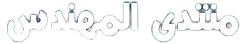# Qualitative Risk Analysis Example

## What Is The Risk Analysis?

When you finish identifying the risks that could affect your construction project, you need to determine which of these risk you will spend time & money on.

Risk analysis is the process of prioritizing all risks based on the probability of the risk occurring & the impact it would have on your project.

## The Difference Between Qualitative and Quantitative Risk Analysis

Project managers should always do qualitative risk analysis because it is quicker than the quantitative risk analysis. Quantitative risk analysis is optional but merited in some cases.

There are 2 primary methods of risk analysis you can use on any construction project :

• Qualitative Risk Analysis
• Quantitative Risk Analysis

The difference between these 2 methods of risk analysis is that qualitative risk analysis uses a relative or descriptive scale to measure the probability of risk to occur whereas quantitative risk analysis uses a numerical scale.

## How to Perform a Qualitative Risk Analysis

There are many ways to perform a qualitative risk analysis. These techniques of analysis require varying degrees of discipline & time.

If the project is small, project managers may use what I call the KISS (Keep It Super Simple) Method . This one-dimensional technique involves rating risks as:

• Very Low
• Low
• Medium
• High
• Very High

The common method is the probability/impact matrix . This two-dimensional technique is used to rate probability & impact. Probability is the likelihood that a risk will occur.

The impact is the consequence or effect of the risk, normally associated with the project objectives such as cost, schedule, scope, and quality.

Rate probability & impact on a scale such as 1 to 5 where 5 is the highest probability and impact. Then we multiply probability times the impact to calculate our risk score. For example, we could rate risk as a probability of 4 & an impact of 3. So risk score would be 4 x 3 = 12.

The scale may be applied to both threats & opportunities. Higher risk scores for threats indicate negative impacts such as adverse impacts on the schedule or budget. And higher risk scores for opportunities indicate positive impacts such as a reduction in the schedule or budget.

## When Should You Perform Qualitative Risk Analysis?

Project managers should facilitate risk evaluation processes early in projects. Throughout the project, risk reviews can be conducted. Current risks are reviewed again & new risks are identified & analyzed.

التحميل
qualitative-risk-analysis.pdf (413.9% u)

المصدر